# 2. Įpp. 492, Marsden & Hoffman Let y : [a,b] → R and ψ : R → R be continuous. Show that A = {(x,o(x)) : x [a,아 C R2 has volume zero in R2 and the set B-{(x, ψ (x)) : x E R} C R2 has measure zero...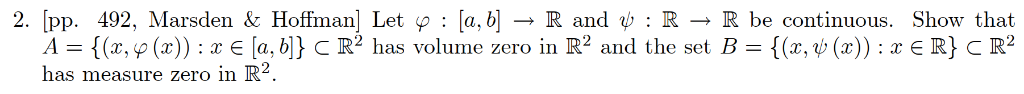2. Įpp. 492, Marsden & Hoffman Let y : [a,b] → R and ψ : R → R be continuous. Show that A = {(x,o(x)) : x [a,아 C R2 has volume zero in R2 and the set B-{(x, ψ (x)) : x E R} C R2 has measure zero in IK.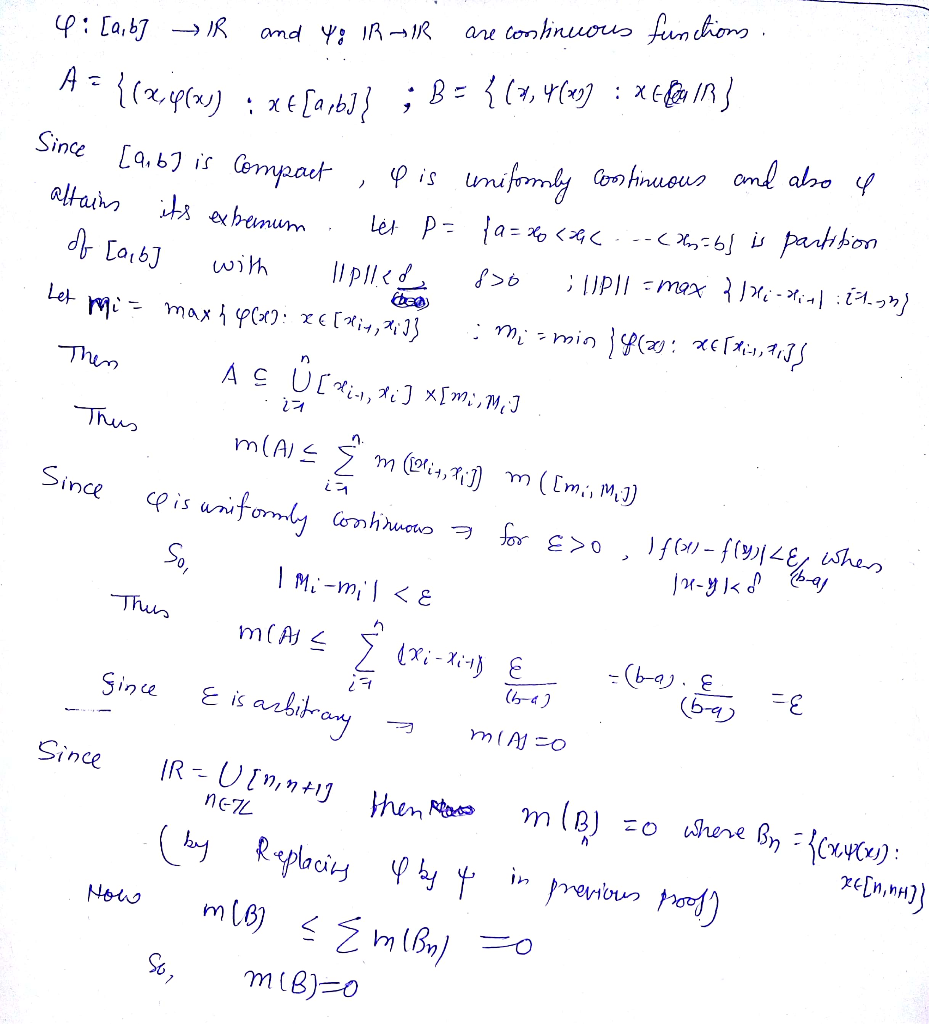##### Add Answer of: 2. Įpp. 492, Marsden & Hoffman Let y : [a,b] → R and ψ : R → R be continuous. Show that A = {(x,o(x)) : x [a,아 C R2 has volume zero in R2 and the set B-{(x, ψ (x)) : x E R} C R2 has measure zero...
Similar Homework Help Questions
• ### 2. [pp. 492, Marsden & Hoffman] Let p [a, bR and :R R be continuous. Show that R} C R2 /-{(z, p (...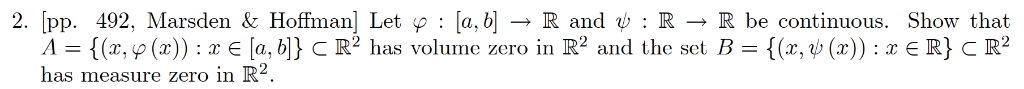2. [pp. 492, Marsden & Hoffman] Let p [a, bR and :R R be continuous. Show that R} C R2 /-{(z, p (x)) : x e [a, b]} C R2 has volume zero in R2 and the set B-{(x, ψ(x)) : x has measure zero in R ie set F 2. [pp. 492, Marsden & Hoffman] Let p [a, bR and :R R be continuous. Show that R} C R2 /-{(z, p (x)) : x e [a, b]} C R2...

• ### Let f : [0, 1] + R be uniformly continuous, so that for every e >...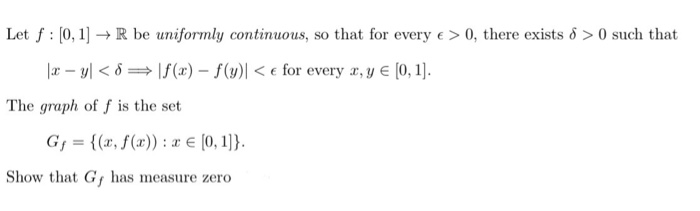Let f : [0, 1] + R be uniformly continuous, so that for every e > 0, there exists 8 >0 such that |- y<f(x) - f(y)<for every x, y € (0,1). The graph of f is the set G = {(x, f(x)) : 2 € (0,1]}. Show that G, has measure zero

• ### Let f : [0, 1] + R be uniformly continuous, so that for every e >...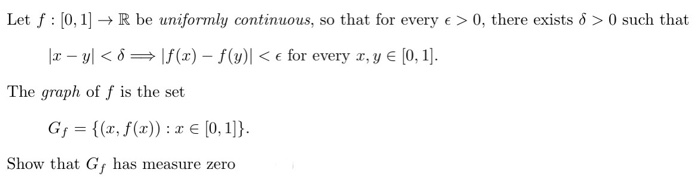Let f : [0, 1] + R be uniformly continuous, so that for every e > 0, there exists 8 >0 such that 12 - y<88\f(x) - f(y)] <e for every x, y € (0,1). The graph of f is the set G= {(x, f(x)) : x € [0,1]}. Show that G has measure zero

• ### 3 This problem is to prove the following in the precise fashion described in class: Let O C R2 be...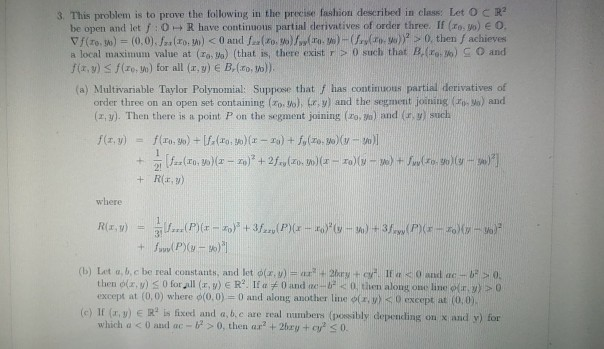Please write carefully! I just need part a and c done. Thank you. Will rate. 3 This problem is to prove the following in the precise fashion described in class: Let O C R2 be open and let f: 0+ R have continuous partial derivatives of order three. If (ro, o) O a local maximum value at (To, Va) (that is, there exist r > 0 such that B. (reo) O and (a) Multivariable Taylor Polynomial: Suppose that f has...

• ### Let B C R" be any set. Define C = {x € R" | d(x,y) <...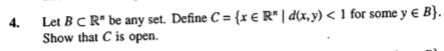Let B C R" be any set. Define C = {x € R" | d(x,y) < 1 for some y E B) Show that C is open.

• ### Let f : R2 → R be a uniformly continuous function and assume that If(y,t)| M. Let yo E R. The goal of this exercise...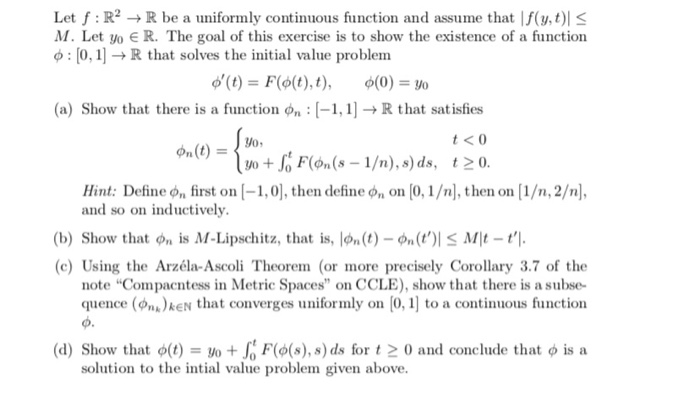Let f : R2 → R be a uniformly continuous function and assume that If(y,t)| M. Let yo E R. The goal of this exercise is to show the existence of a function φ : [0, 1] → R that solves the initial value problem o'(t)-F(d(t),t), ф(0)-Yo (a) Show that there is a function n1,R that satisfies t <0 n(リーレ0+.GF(du(s-1/n),s)ds, t20. Hint: Define фп first on [-1,0] , then define фп。n [0,1 /n), then on [1/n, 2/n], and so on...

• ### Let the universal set be R, the set of all real numbers, and let A {xE...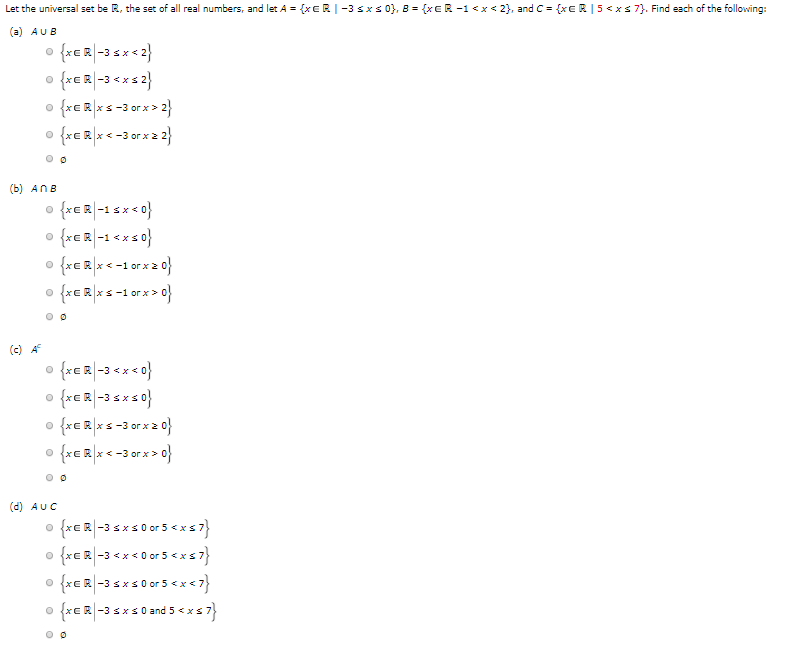Let the universal set be R, the set of all real numbers, and let A {xE R I -3 sxs 0, B {xER -1< x 2}, and C xE R | 5<xs 7}. Find each of the following: (a) AUB {xR-3 < x2} s -3orx > 과 xs. (b) AnB xR-12 {*E찌-1 <xs마 frER< -1 orx {*ER|x s -1 or*> 아 (c) A {*ER-3 <x< 아} {*ER|-3 < 아} s-3 orx> 아 frER< 3 orx x s 0 (d) AUC...

• ### Implicit Function Theorem in Two Variables: Let g: R2 → R be a smooth function. Set {(z, y) E R2 ...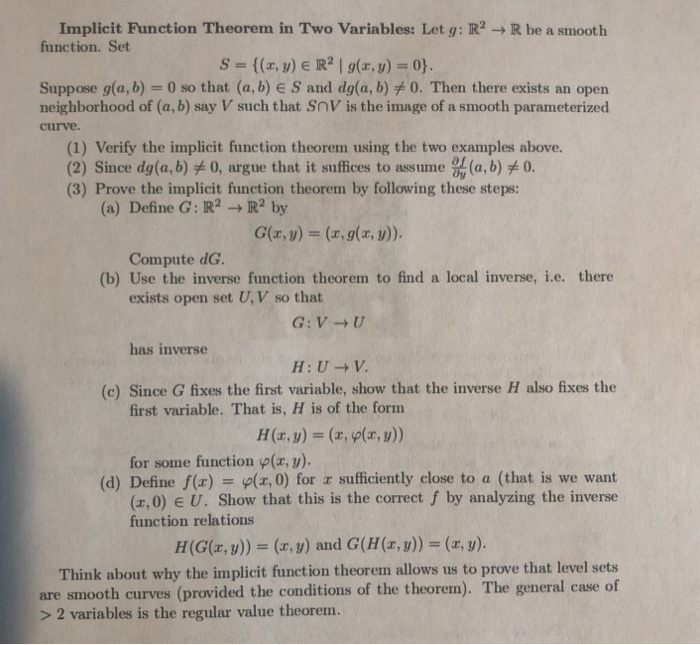Implicit Function Theorem in Two Variables: Let g: R2 → R be a smooth function. Set {(z, y) E R2 | g(z, y) = 0} S Suppose g(a, b)-0 so that (a, b) E S and dg(a, b)メO. Then there exists an open neighborhood of (a, b) say V such that SnV is the image of a smooth parameterized curve. (1) Verify the implicit function theorem using the two examples above. 2) Since dg(a,b) 0, argue that it suffices to...

• ### Let f : [0,1] → R be uniformly continuous, so that for every e > 0,...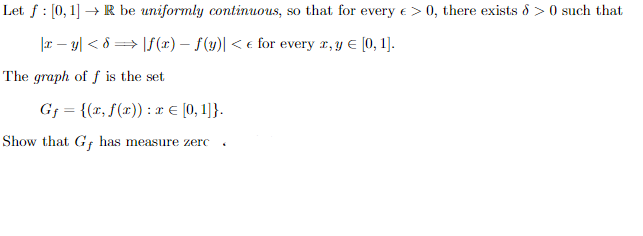Let f : [0,1] → R be uniformly continuous, so that for every e > 0, there exists 8 >0 such that 2 - y<== f() - f(y)< € for every 2, Y € [0,1]. The graph of f is the set Gj = {, f(c)): 1 € [0,1]}. : Show that G has measure zero.

• ### Let f : [0,1] → R be uniformly continuous, so that for every e > 0,...Let f : [0,1] → R be uniformly continuous, so that for every e > 0, there exists 8 >0 such that 2 - y<== f() - f(y)< € for every 2, Y € [0,1]. The graph of f is the set Gj = {, f(c)): 1 € [0,1]}. : Show that G has measure zero.

Free Homework App